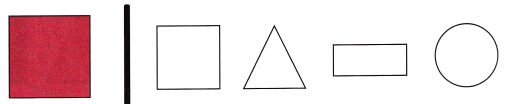# McGraw Hill Math Grade 1 Chapter 11 Lesson 3 Answer Key Shapes and Size

All the solutions provided in McGraw Hill Math Grade 1 Answer Key PDF Chapter 11 Lesson 3 Shapes and Size as per the latest syllabus guidelines.

## McGraw-Hill Math Grade 1 Answer Key Chapter 11 Lesson 3 Shapes and Size

Identify

Find the shape that matches. Color it blue.

Question 1.Explanation:
The given shape is a circle so, colored  the circle shape.
A circle has curved surface around a center point.

Question 2.Explanation:
The given shape is a triangle so, colored the triangle.
A triangle has 3 sides and 3 corners.

Question 3.